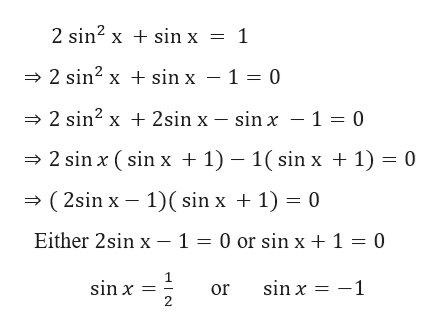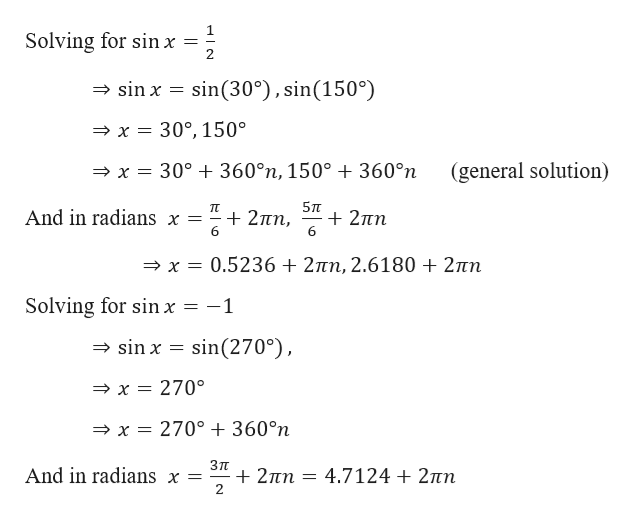# Solve the equation (x in radians and θ in degrees) for all exact solutions where appropriate. Round approximate answers in radians to four decimal places and approximate answers in degrees to the nearest tenth.2 sin2 x + sin x = 1

Question
14 views

Solve the equation (x in radians and θ in degrees) for all exact solutions where appropriate. Round approximate answers in radians to four decimal places and approximate answers in degrees to the nearest tenth.

2 sin2 x + sin x = 1

check_circle

Step 1

Given,help_outlineImage Transcriptionclose2 sin2 x sin x = 1 2 sin2 xsin x - 1 0 2 sin2 x2sin x - sin x -1 = 0 2 sin x (sin x + 1) -1( sin x 1) 0 (2sin x 1) sin x 1) 0 Either 2sin x - 1 = 0 or sin x + 1 = 0 sin x 1 sin x fullscreen
Step 2

Further calcu...help_outlineImage TranscriptioncloseSolving for sin x sin(30°), sin(150°) sin x = x = 30°,150 x = 30° +360°n, 150° 360°n (general solution) 5п 2n 6 TT And in radians x = 27n 6 x= 0.5236 +27tn, 2.6180 2n Solving for sin x = -1 sin x sin(270) x 270° 270° + 360°n x Зп +2пп 2 And in radians x = = 4.7124 2tn fullscreen

### Want to see the full answer?

See Solution

#### Want to see this answer and more?

Solutions are written by subject experts who are available 24/7. Questions are typically answered within 1 hour.*

See Solution
*Response times may vary by subject and question.
Tagged in

### Trigonometric Ratios